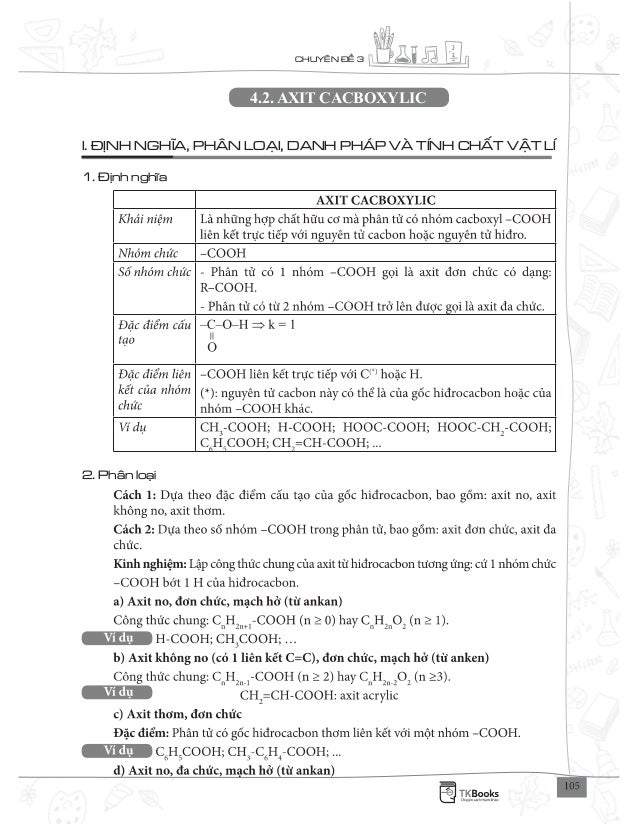Successfully reported this slideshow.Upcoming SlideShare
×

# But pha diem thi thptqg hoa huu co ban doc thu

2,001 views

Published on

Bản đọc thử sách Bứt phá điểm thi THPT Quốc gia môn Hóa - Phần Hóa hữu cơ.

Published in: Education
• Full Name
Comment goes here.

Are you sure you want to Yes No• Be the first to comment

### But pha diem thi thptqg hoa huu co ban doc thu

1. 1. CHUY N 3 4 2 AX T CACBOXYL C I. NH NGH A, PHÂN LOẠI, DANH PHÁP VÀ T NH CHẤT VẬT L 1. nh ngh a 2. Ph n lo i
2. 2. CHUY N : AN EHIT - AXIT CACBOXYLIC 3. Danh ph p (no; n ch c; m ch h ) 4. Tnh ch t v t l II. T NH CHẤT HOÁ H C 1. Tnh axit y u
3. 3. CHUY N 3 2. Ph n ng th nh m – OH (ph n ng este h a) 3. Ph n ng t ch y 3n 2 2 − 0 t 2 2CO H On n= 2 2CO H On n= 0 t 0 t 4. Ph n ng ri ng c a m t s axit cacboxylic - OH o t III. I U CH VÀ NG D NG 1. i u ch men giaám xt 0 xt 180 C, 50 atm
4. 4. CHUY N : AN EHIT - AXIT CACBOXYLIC 0 t , xt 2. ng d ng B. PH NG PHÁP GIẢI BÀI TẬP π
5. 5. CHUY N 3 2.2n 3n 2 k 0,5n 1 2 − + = = +
6. 6. CHUY N : AN EHIT - AXIT CACBOXYLIC 2.3 6 2 k 1 2 − + = = − − −3CH CH CH CHO | | OH Cl
7. 7. CHUY N 3 II.PHẢN NGC NGH2 ,Br2 VÀOCH C–CHOVÀLI NK Tπ(C=C) π π 2 2 BTKL H ancol X Hm m m 3,1 2,9 0,2 (gam) n 0,1 (mol)= − = − = = A 2,9 M 29 (CHO) 0,1 = =
8. 8. CHUY N : AN EHIT - AXIT CACBOXYLIC 2A H A 1 2,9 n .n 0,05 M 58 (HOC-CHO) 2 0,05 = = = = n∆ n∆ V∆
9. 9. CHUY N 3 π 0 t , xt n 2n 2 2k x 2 n 2n 2 x 2x x C H CHO k x H C H CH OH+ − − + −+ + C, C π BTKL 9 4,5 (gam) 2 = 2 2 2CO H O CO 4,5 n n 0,25 (mol) V 0,25.22,4 5,6 (l) 18 = = = = =
10. 10. CHUY N : AN EHIT - AXIT CACBOXYLIC BTKL Y Xm m 2.4,7.4 37,6 (gam)= = = Y Y Y m 37,6 n 1 (mol) M 9,4.4 = = = n∆ 2ancol X Hn n n 1 (mol)= = = 2 2H ancol H 1 n .n 0,5 (mol) V 11,2 (l) 2 = = = Ch 2 Ph n ng AgNO3 à h CHO à l n k t ba o t o t R C CH−
11. 11. CHUY N 3 16 %mO .100% 53,33% 30 = = 2 4 16.2 %mO .100 37,21 R 28 (C H ) R 29.2 = = = + ancol 3 M 46 CH OH< nAg 0,4 m 43,2 (g)= = 3 32 5 2 5AgNO /NH BTKL HCHOBTNT.Ag C H C CH : x (mol) C H C CAg : x (mol) 13,8 (g) HCHO: y (mol) Ag : 4y (mol) 54x 30y 13,8 x 0,2 30.0,1 %m .100 21,74% y 0,1 13,8x 4y 0,6 +− − + = = = = =+ =
12. 12. CHUY N : AN EHIT - AXIT CACBOXYLIC Ag 43,2 n 0,4 (mol) 108 = = 2H+ Na+ 0,1 mol 12 gam o t , xt 3 3AgNO /NH BTNT.C Ag Ag : 2x 4y (mol) 1,8 x y 0,06 x 0,045 (mol) x 0,04530 H% .100 .100 75% 16,2 y 0,015 (mol) 0,06 0,06 n 2x 4y 0, HCOOH : x (mol 15 ) HCHO: y (mol) 108 + + + = = = = = = = = + = =
13. 13. CHUY N 3 3 3AgNO /NH+ Ag2 1 Ag n (2)m 3,2 0,8 m n (1) 4 = = = 1. L m qu tm h a 2. T c d ng v i kim lo i 1 2 2H axit 2.n x = n 2 BTNT.H H( COOH) Hn 2.n− =